# Constructions

Geometric Constructions ... Animated!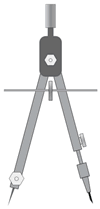"Construction" in Geometry means to draw shapes, angles or lines accurately.

These constructions use only compass, straightedge (i.e. ruler) and a pencil.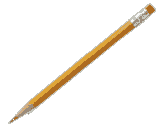This is the "pure" form of geometric construction: no numbers involved!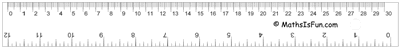Learn these two first, they are used a lot: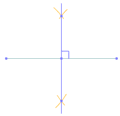Line Segment Bisector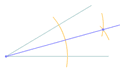Angle Bisector And it is useful to know how to do 30°, 45° and 60° angles. We can use the angle bisector method (above) to create other angles, such as 15°, etc: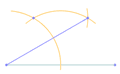30 Degree Angle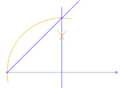45 Degree Angle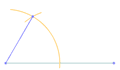60 Degree Angle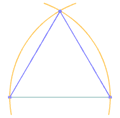Equilateral Triangle Points and Lines: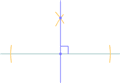Perpendicular to a Point on a Line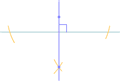Perpendicular to a Point NOT on a Line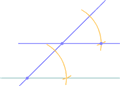Parallel Line through a Point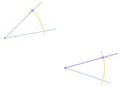Same (Congruent) Angle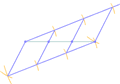Cut a line into N segments Circles: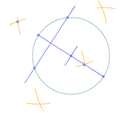Center of a Circle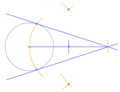Point to Tangents on a Circle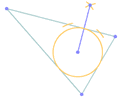Inscribe a Circle in a Triangle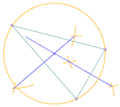Circumscribe a Circle on a Triangle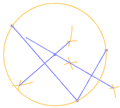Circle touching 3 Points And for the "Master Class", a Pentagon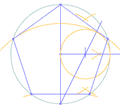Pentagon

(Note: You can also see how to Use the Protractor, Use the Drafting Triangle and Ruler, and How to construct a Triangle With 3 Known Sides, but they are not "pure" geometric constructions.)